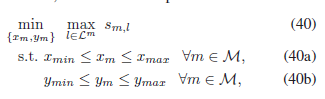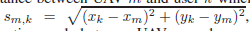# CVX Matlab code For Optimization Problem

Hello everyone!
Can you help me with the problem in CVX.

The above problem is a convex optimization problem and can be solved by using optimization tools such as CVX . the 2D coordinates (xm, ym) of UAV m are obtained.You can use `norm` for s_{m,k} .

This looks very straightforward. Please read the CVX Users’ Guide and try yourself. You will need to declare `s` as an expression holder (array), per http://cvxr.com/cvx/doc/basics.html#assignment-and-expression-holders .

Then let us know if you encounter any stumbling blocks.

clear ;
clc;
close all;
%X=zeros(2,1000);
%Xm=zeros(2,1);
xmin=0;
ymin=0;
xmax=6000;
ymax=6000;

Xl = randi(6000, 1, 200);
Yl = randi(6000, 1, 200);
plot(Xl,Yl, ‘^’, ‘MarkerSize’, 5, ‘LineWidth’, 3)
hold on

%Xm = randi(6000, 1, 30);
%Ym = randi(6000, 1, 30);
%plot(Xm, Ym, ‘rs’, ‘MarkerSize’, 5, ‘LineWidth’, 4)
%hold on
%grid on

for Xm = 1:30
for Ym = 1:30
Sml = sqrt((Xl-Xm).^2 + (Yl-Ym).^2);
end
end

%Sml = sqrt((Xl-Xm)^2 + (Yl-Ym)^2);

cvx_begin
variables Xm Ym

``````minimize norm( Sml )

subject to

xmin <= Xm <= xmax;
ymin <= Ym <= ymax;
``````

cvx_end

I corrected the code, it was solved. is it right?

No, it’s not.

You need to declare `s` as an expression after the variable declarations for Xm and Ym, which need to be declared as arrays,. Then you need to assign the values to `s`. The objective must be after that, and is to minimize the max of suitable indexed s`. Your code as it is now, is minimizing a constant, i.e., really just a feasibility problem, and produces whatever scalar value of Xm and Ym that the solver chooses “at its whim”. I.e., not much relation to your problem.

I suggest, you re-read the CVX Users’ Guide, and start with simple examples in there, and then try to make modifications to those examples. Get good at that before trying to progress to your current problem. And make sure that you understand the problem specification including what all the indexing means.

Is this code the answer to this problem?

code:
clc;
clear all;
close all;
%% start simulation
L_area = 6000; % m
xmin=0;
ymin=0;
xmax=L_area;
ymax=L_area;
L = 200; % The number of users
User_coordinates=randi(L_area, 2, L);
M = 10; % The number of drons
User_S = reshape(User_coordinates,2,[],M);
X_Find=zeros(1,M);
Y_Find=zeros(1,M);
for u=1:M
cvx_begin ‘quiet’
variables xm ym
for i=1:size(User_S,2)
s_ml(i) = (xm-User_S(1,i,u)).^2+(ym-User_S(2,i,u)).^2;
end
minimize(max(s_ml))
subject to
xmin <= xm <= xmax;
ymin <= ym <= ymax;
cvx_end
X_Find(1,u) = xm;
Y_Find(1,u) = ym;
clear s_ml
end
disp(‘The solution is’)
X_Find
Y_Find

It might be sort of correct, but has some things which could cause difficulties.

When run as is, Mosek declared it infeasible, although other solver didn’t. Perhaps that is due to the very large objective function values. That can be improved by instead using the norm, as the formulation in the image uses.
`s_ml(i) = norm([xm-User_S(1,i,u);ym-User_S(2,i,u)]);`
Then Mosek solves it without difficulty.

It’s also probably a good idea to change units, for instance from meters to km. That will improve the scaling of the problem, and make solvers less likely to have difficulty.

You seem to be getting away with not having the statement
`expression s_ml(size(User_S,2))` prior to the for loop in which `s_mi(i)` is set. I recommend you include it though, as constituting good practice whenever you assign individual elements of an expression which is not a scalar.

I also recommend you don’t use `quiet` until you have verified everything is working correctly.